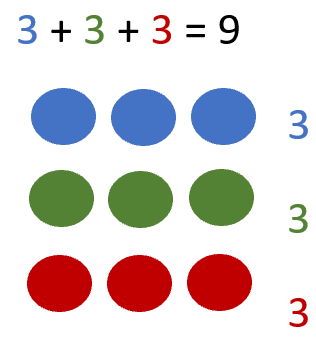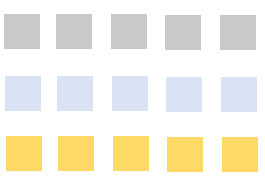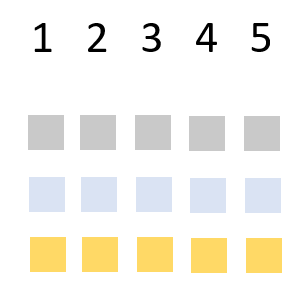# Math Worksheets Land

Math Worksheets For All Ages

# Math Worksheets Land

Math Worksheets For All Ages

Two easy ways to count objects in rows and columns - Counting objects is all fun and games until you get too many things to count. Like, imagine you in your teacher's shoes, who have to count you and all your classmates every day. So, now, we will discuss easier ways to count objects present in rows and columns. 1. By multiplication, we can count objects quickly and correctly. Let's suppose you have 3 rows, where you and your class-fellows sit in the classroom. Each row has 10 students sitting right behind each other. So, you can just multiply 3 with 10, and you will find the total number of students in your class without counting all of them one by one. 2. Take an array of numbers, and let us consider the previous example again in a different way. You are sitting in a pair of three in each row. There are three pairs in total, and you have 10 pairs in the whole classroom. So, now we multiply 3 by 10, and you will get 30. 30 are the total students in your classroom. With these math worksheets we have students actively learn to use rows and columns in math.

### Aligned Standard: Grade 2 Operations - 2.OA.4

• Answer Keys - These are for all the unlocked materials above.

### Homework Sheets

We use visuals, outlines, and number in the different arrays to work through.

• Homework 1- In a shop, there are some cookies arranged in two rows with seven columns. Each cookies is used by only one student. What is the total number of students in the class?
• Homework 2- Write an addition sentences based on the blocks.
• Homework 3- Write the addition sentence that is shown below.

### Practice Worksheets

Every problem will offer you a new unique perspective.

• Practice 1- Sherry bought some new skirts. She arranges the skirts in her closet. She arranged them in four rows with four columns. How many skirts did she bring home?
• Practice 2- Write an addition sentence to match the block pattern below.
• Practice 3- James bought some new toys. He has to arrange the toys in his closet. He arranged them in three rows with three columns. How many toys did he bring home?

### Math Skill Quizzes

Quizzes were a little tough to put together because each problem takes up a lot of space.

• Quiz 1- Ana bought new gowns. She has to arrange them in her closet. She arranged them in four rows with four columns.
• Quiz 2- Peter brought new masks to his home. He has to arrange the masks in his closet. He arranged them in five rows with five columns. How many masks did he bring home?
• Quiz 3- Write an addition sentences for the blocks.

### What Are Arrays in Math?

An array is a visual that can be used to help students learn how represent and eventually perform multiplication and division. They can also be a great jump off point for learning how to count and transition that skill into addition and subtraction operations. It is a series of shapes (often circles) that are arranged into row and columns. The shapes are used often to help students solve word problems, but they can be used as a supplement to tape diagrams and number bonds. When writing an array, it is often important to understand the different significance of row versus that of the column. Rows are used to signify groups and are drawn horizontally (left to right). Columns are used to signify the number in each group and are drawn vertically (top to bottom). For counting arrays help students easily transition for counting in sequential order to skip counting. You just group the objects in each row/column to match the value you are skipping by. This can also be used to ease your mindset to processing math operations. This simple visual can be a powerful tool to learn the concept of multiplication. Student can easily start to physically see the values directly in front of them.

## Why Are 2nd Grade Arrays So Important?

At the 2nd grade level, we are focused on using this strategy to help us solve addition problems. While this does not seem like a mind-blowing technique, there is a deeper purpose of using this technique in the hopes that it will be a quick revisit before we can use when multiplication and division operations become more common place. Understanding those higher-level operations is much easier using this technique. If students become comfortable using this technique now, it will be an easier pivot to using them at those higher levels.

There are 2 primary skills that we work on here. Both involve visualizing and interpreting values that undergoing a sum. Here is an example of taking a visual and creating an equation for it. Draw an array for the equation: 3 + 3 + 3 = 9

We would begin by choosing a consistent visual for each number. In this case, we chose the shape of a circle. We would create a row and use 3 circles to represent the first value (3). We then create our second row to represent the second value (3), to show that this is a second value, we change the color of the circles (to green). We then create our third row to represent the third value (3), to show that this is another unique value, we change the color of the circles (to red).The other method that applies to using 2ng grade arrays in sums is determining an equation that is depicted by a visual array. This is basically working backwards from the method we just learned. Write an addition equation to depict the array found below.This array has 3 different types of the same data. We can tell this because of the three different colors (gray, blue, gold) that are used. We start by counting the number of squares found in each column.In this case we see that each of three different values is equal to a value of 5. This tell us that this array can be defined by the equation: 5 + 5 + 5 = ____. We can either complete the sum or just add all the squares up to find that the final equation should be: 5 + 5 + 5 = 15

Unlock all the answers, worksheets, homework, tests and more!
Save Tons of Time! Make My Life Easier Now

## Thanks and Don't Forget To Tell Your Friends!

I would appreciate everyone letting me know if you find any errors. I'm getting a little older these days and my eyes are going. Please contact me, to let me know. I'll fix it ASAP.